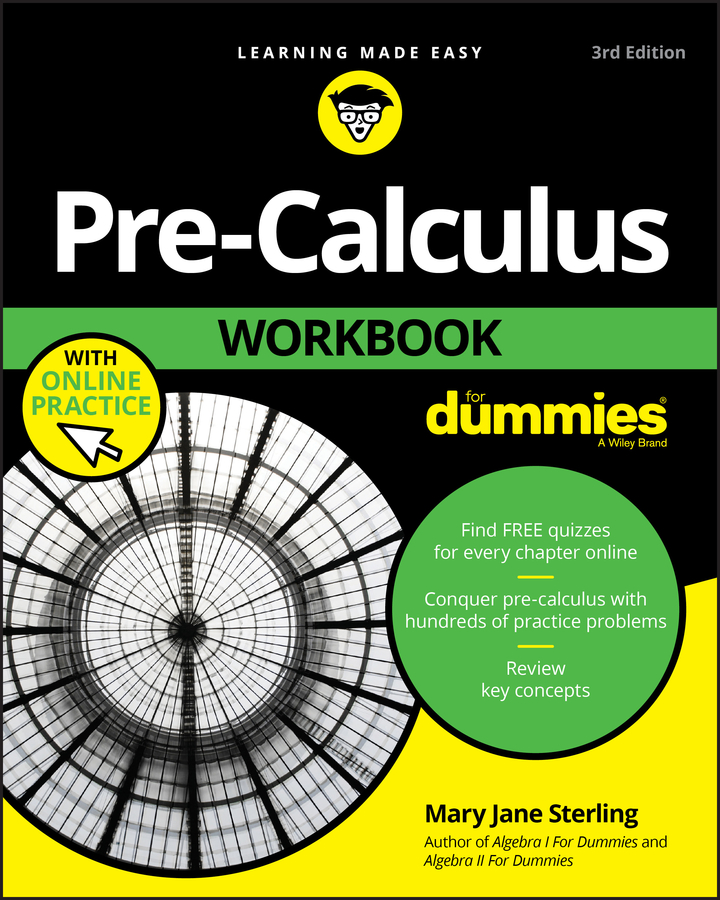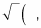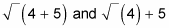##### Pre-Calculus Workbook For DummiesIt’s a good idea to purchase a graphing calculator for pre-calculus work. Since the invention of the graphing calculator, math classes have begun to change their scope. A graphing calculator does so many things for you, and even if a teacher won’t allow you to use one on a test, you can almost always use one to check your work on homework problems.

Many different types of graphing calculators are available, and their individual inner workings are all different. To figure out which one to purchase, ask advice from someone who has already taken a pre-calculus class, and then look around on the Internet for the best deal.

Just a hint: If you can find one with an exact/approximate mode, it’s worth the investment because it will give you exact values (rather than decimal approximations), which is often what teachers are looking for.

If by chance you’re allowed to use a graphing calculator, you should still do the work by hand. Then use your graphing calculator to check your work. This way you won’t become dependent on technology to do work for you; someday, you may not be allowed to use one (on a college math-placement test, for example). It is also wise to understand how to do a problem by hand so that you know if the solution your calculator gives you makes sense. If you punch in the wrong numbers into a calculator by accident, then you will be given the wrong answer. Knowing the theoretical concepts behind pre-calculus helps you spot these errors!

Many of the more theoretical concepts in pre-calculus are lost when you use your graphing calculator. Whether you’re allowed to use the graphing calculator or not, be smart with its use. If you plan on moving on to calculus, you need to know the theory and concepts behind each topic.

• Always double check that the mode in your calculator is set according to the problem you’re working on. Look for a button somewhere on the calculator that says mode. Depending on the brand of calculator, this button allows you to change things like degrees or radians, or f(x) or r(i). For example, if you’re working in degrees, you must make sure that your calculator knows that before you ask it to solve a problem. The same goes for working in radians. Some calculators have more than ten different modes to choose from. Be careful!

• Make sure you can solve for y before you try to construct a graph. You can graph anything in your graphing calculator as long as you can solve for y. The calculators are set up to accept only equations that have been solved for y.

• Be aware of all the shortcut menus available to you and use as many of the calculator’s functions as you can. Typically, under your calculator’s graphing menu you can find shortcuts to other mathematical concepts (like changing a decimal to a fraction, finding roots of numbers, or entering matrices and then performing operations with them). Each brand of graphing calculator is unique, so read the manual. Shortcuts give you great ways to check your answers!

• Type in an expression exactly the way it looks, and the calculator will do the work and simplify the expression. All graphing calculators do order of operations for you, so you don’t even have to worry about the order. Just be aware that some built-in math shortcuts automatically start with grouping parentheses.

For example, a calculator may start a square root off asso all information you type after that is automatically inside the square root sign until you close the parentheses. For instance,represent two different calculations and, therefore, two different values (3 and 7, respectively). Some smart calculators even solve the equation for you. In the near future, you probably won’t even have to take a pre-calculus class; the calculator will take it for you!